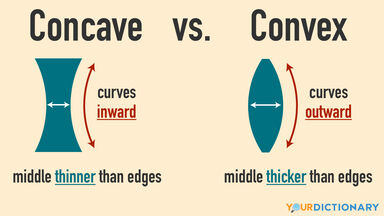# Concave vs. Convex: Basic Differences to Know

It's important to know the difference between concave vs. convex because the two words have opposite meanings. Getting these terms mixed up in your writing can result in significant misunderstanding. Master the basic differences so you can use the words concave and convex in your vocabulary with confidence.infographic concave versus convex shapes

## Concave vs. Convex

The words concave and convex come up in many math and science classes. These two words are closely related to one another. Both words can be used as a noun or as an adjective. They differ in that each word describes a specific shape. A concave shape is the opposite of a convex shape.

The terms concave and convex are most commonly used as adjectives. When used as an adjective, these words describe the shape of an object, by specifying whether the object curves inward or outward.

• An object with a concave shape curves inward, such as a spoon or a bowl. The middle is thinner than the edges.
• An object with a convex shape is one that curves outward, such as a basketball or a baseball. The middle is thicker than the edges.

The words concave and convex describe the direction that an object curves. Because concave shapes curve in and convex shapes curve out, each of these two words describes opposite shapes.

### Noun Usage

The words concave or convex can be used as nouns. When used this way, the terms will be used to name specific things rather than being used to describe the shape of another object.

• A concave is any line or surface that curves inward. (This line is a concave.)
• A convex is any line or surface that curves outward. (This surface is a convex.)

It is not as common to use concave or convex this way. It is much more common to use these terms as adjectives.

## How to Remember the Difference Between Concave and Convex

Remembering which shape (convex or concave) curves in can be challenging because the two terms sound so similar. In order to help make sure that you remember which word represents which shape, use this simple trick.

• If something caves-in, that means it falls inward. Concave has the word cave in it.
• Remember, a cave-in goes inward, so that means a concave shape must curve in.
• Convex has ex in it. Ex is the beginning of the word exit. When you exit, what do you do? You go out.
• Associate the ex in convex with exiting. This can help you remember that a convex shape curves out.

## Concave and Convex in the Real World

There are several examples of concave and concave shapes in the real world. From trips to the amusement park to eyeglasses to correct your vision, the concepts of concave and convex impact many aspects of daily life. That's one reason why it's so important to use these terms correctly.

### Concave vs. Convex Mirrors

If you've ever stood in front of a mirror that made you appear a different size than what you really are, you have experienced either convex or concave mirrors in action, or maybe even both. Funhouses at amusement parks often have both kinds of mirrors to amaze visitors by how different their image can look from one mirror to another.

• Mirrors that make you look smaller than you really are are convex mirrors. This type of mirror curves outward.
• Mirrors that make you look larger than you really are are concave. Look closely and you'll see that this type of mirror curves inward.

### Concave vs. Convex in Spinal Curves

Rather than being straight up and down, a person's spine (backbone) has curves in it. Not everyone's spine has identical curves, as there are several factors that can impact exactly where and how a person's spine curves. However, a spine does have both concave and convex curves.

• Where a person's spine curves inward, toward the front of the individual's body, that is a concave curve.
• Where a person's spine curves outward, away from his or her front side, that is a convex curve.